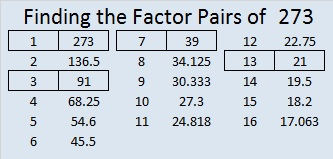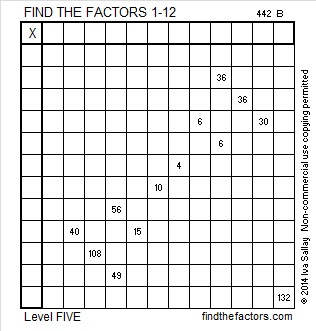# 273 and Level 5

• 273 is a composite number.
• Prime factorization: 273 = 3 × 7 × 13
• The exponents in the prime factorization are 1 and 1. Adding one to each and multiplying we get (1 + 1)(1 + 1)(1 + 1) = 2 × 2 × 2= 8. Therefore 273 has exactly 8 factors.
• Factors of 273: 1, 3, 7, 13, 21, 39, 91, 273
• Factor pairs: 273 = 1 × 273, 3 × 91, 7 × 39, or 13 × 21
• 273 has no square factors that allow its square root to be simplified. √273 ≈ 16.523Find the Factors is now one of  many  interesting mathematical resources that can be found on Solvemymaths!Print the puzzles or type the factors on this excel file: 12 Factors 2014-10-20